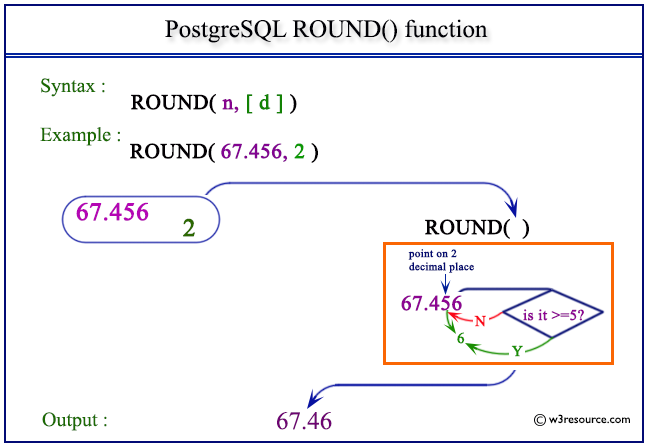# PostgreSQL ROUND() function

## ROUND() function

The PostgreSQL round() function is used to return the value after rounded a number upto a specific decimal places, provided in the argument.

Syntax:

```random()
```

PostgreSQL Version: 9.3

Pictorial presentation of PostgreSQL ROUND() functionExample 1: PostgreSQL ROUND() function

Code:

``````SELECT ROUND(67.456) AS "Round";
```
```

Sample Output:

``` Round
-------
67
(1 row)
```

Example 2: PostgreSQL ROUND() function

Code:

``````SELECT ROUND(67.456,1) AS "Round upto 1 decimal";
```
```

Sample Output:

``` Round upto 1 decimal
----------------------
67.5
(1 row)
```

Example 3: PostgreSQL ROUND() function

Code:

``````SELECT ROUND(67.456,2) AS "Round upto 2 decimal";
```
```

Sample Output:

``` Round upto 2 decimal
----------------------
67.46
(1 row)
```

Previous: RANDOM function
Next: SIGN function

﻿